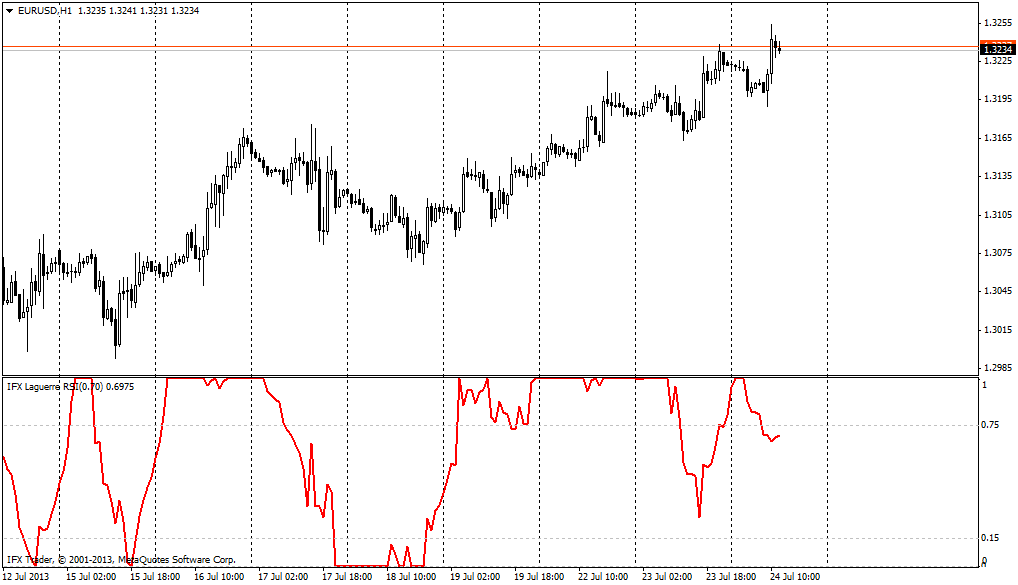#### 公式

L0:=((1-g)*C) + (g*PREV);

L1:=(-g*L0) + Ref(L0,-1) + (g*PREV);

L2:=(-g*L1) + Ref(L1,-1) + (g*PREV);

L3:=(-g*L2) + Ref(L2,-1) + (g*PREV);

cu:= If(L0 > L1, L0-L1,0) + If(L1 > L2, L1-L2,0) + If(L2 > L3, L2-L3,0);

cd:= If(L0 < L1, L1-L0,0) + If(L1 < L2, L2-L1,0) + If(L2 < L3, L3-L2,0).

#### 应用

MetaTrader平台的RSI拉盖尔指数的主要参数是伽玛因子，其默认值是0.7。需要注意的是当其取值小于该水平时，（例如0.5）该指标会产生大量建议积极交易的信号，这对交易结果会产生负面影响。指标因子的取值过高（大于0.85）也是不明智的，因为过大的取值或会导致该指标反应滞后。

RSI拉盖尔指标的相对独特的特点是当指标线在最小或最大值处且严格按照水平方向延伸，就意味着强有力的价格趋势。因此，如果指标等于一且水平运动，则价格牛势会弥漫整个市场。如果该指标等于零且水平运动，则应在正在发展中的下跌趋势中做空。gamma = 0.7